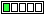All about flooble | fun stuff | Get a free chatterbox | Free JavaScript | Avatarsperplexus dot infoAge Ascertainment II (Posted on 2013-02-01)```       +-----+-----+
|  A  |     |
+-----+-----+-----+
|  B  |     |
+-----+-----+
```
The ages of Ambrose, Brandon and Chester can be related to the diagram above so that when just one digit is written in each box:
1. A across is Ambrose's age in years.
2. A down is the sum of Ambrose's and Brandon's age in years.
3. B across is the sum of Ambrose's age, Brandon's age and Chester's age in years.
4. Two of Ambrose, Brandon and Chester are the same age in years.
Who is a different age in years from the other two?

 No Solution Yet Submitted by K Sengupta Rating: 1.0000 (2 votes)Comments: ( Back to comment list | You must be logged in to post comments.)Age Ambiguity (spoiler)| Comment 1 of 4
Observations:

1) Ages of A + B + C end in the same digit as A + B, so C ends in a zero.
2) Ages of A + B start with same digit as A, so B is necessarily 0 through 9.
3) If an Age of 0 is allowed, then B and C could be newborn twins, and A is the odd man out.
4) If an Age of 0 is not allowed, but ages starting with zero are allowed, then C could be odd man out.  For instance, Ambrose = Brandon = 4, and C is 90.
5) So I guess that we are supposed to assume that ages cannot be 0 and cannot start with a zero.  In that case, B is 1 through 9 and cannot be the same as C (which ends in 0).  And B cannot be the same as A, because A is more than 9.  So B is the odd man out.  Possible example: A = 10, C = 10, B = 5.

Alternative answers (depending on assumptions): A, C

 Posted by Steve Herman on 2013-02-01 10:33:53Please log in:

 Search: Search body:
Forums (2)